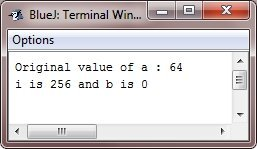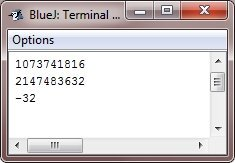# Java left-shift operator

The left-shift operator, <<, simply shifts all of the bits in a value to the left a specified number of times. Here is the general form to use the left-shift operator in Java:

`value << num`

Here, num specifies the number of positions to left-shift the value in value, i.e., the << simply moves all of the bits in the specified value to the left by the number of bit positions specified by num. For each shift left, the high-order bit is simply shifted out (and lost), and a zero is brought in on the right. This means that when a left shift is applied to an int operand, bits are lost once they are shifted past bit position 31. If the operand is long, bits are lost after bit position 63.

When you shift the values of byte and short, Java's automatic type promotions lead to unexpected results.

As you know, byte and short values are promoted to int when an expression is evaluated.

Moreover, the result of such an expression is also an int. This means that the outcome of a left shift on a byte or short value will simply be an int, and the bits shifted left will not be lost until they shift past bit position 31.

Also, when a negative byte or short value is changed to an int, the sign will be extended.Therefore, the high-order bits will be filled with 1's. For these reasons, to perform a left shift on a byte or short implies that you must eliminate the high-order bytes of the int result.

For example, if you left-shift a byte value, that value will first be promoted to an int and then shifted. It means that you must discard the top three bytes of the result when what you want is the result of a shifted byte value. The simplest way to do this is to cast the result back into a byte.

## Java Left Shift Operator Example

Here, this program illustrates the concept of the left-shift operator:

```public class JavaProgram {
public static void main(String args[]) {
byte a = 64, b;
int i;

i = a << 2;
b = (byte) (a << 2);

System.out.println("Original value of a : " +a);
System.out.println("i is " + i + " and b is " + b);
}
}```

When the above Java program is compiled and executed, it will produce the following output:Since a is promoted to int for the purposes of evaluation, left-shifting the value 64 (0100 0000) twice results in i containing the value 256 (1 0000 0000). However, the value in b contains 0, as after the shift, the low-order byte is now zero. Its only one bit has been shifted out.

Since each left shift has the effect of doubling the original value, programmers frequently use this fact as an efficient alternative to multiplying by 2. But you need to watch out. That is, if you shift a bit into the high-order position (bit 31 or 63), the value will become negative. Here is how this program illustrates this:

```public class JavaProgram {
public static void main(String args[]) {
int i;
int num = 0xFFFFFFE;

for(i=0; i<4; i++) {
num = num << 1;
System.out.println(num);
}
}
}```

When the above Java program is compiled and executed, it will produce the following output:The starting value was carefully chosen because, after being shifted left by four bit positions, it would produce the value -32. As you can see, when a bit is shifted in bit 31, the number is interpreted as negative.

### Advantages of the left-shift operator in Java

• Java's left-shift operator performs integer bitwise operations efficiently.
• The left-shift operator speeds up powers of 2 multiplication. For example, "x<<3" is equivalent to "x*8."
• Left shift creates bit masks for bitwise AND operations.

### Disadvantages of the left-shift operator in Java

• If the number being shifted is greater than the maximum value of the target type or the shift amount is greater than or equal to the number of bits in the target type, the result may be unpredictable and lead to loss of precision.
• Only bitwise operations and multiplication by powers of 2 can use the left shift operator. It's not arithmetic.
• For bitwise novices, the left shift operator can be confusing. This makes code maintenance and debugging harder.

Java Online Test

« Previous Tutorial Next Tutorial »